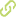# The fibonacci numeral system for computer vision Chapter in Scopus•
• Overview
•
• Identity
•
• Additional Document Info
•
• View All
•

### abstract

• © 2018, Springer International Publishing AG. One of the most important challenges when creating efficient systems of technical vision is the development of efficient methods for enhancing the speed and noise-resistance properties of the digital devices involved in the system. The devices composed of counters and decoders occupy a special niche among the system¿s digital tools. One of the most common ways of creating noise-proof devices is providing special coding tricks dealing with their informational redundancy. Various frameworks make that possible, but nowadays, an acute interest is attracted to noise-proof numeral systems, among which the Fibonacci system is the most famous. The latter helps generate the so-called Fibonacci codes, which can be effectively applied to the computer vision systems; in particular when developing counting devices based on Fibonacci counters, as well as the corresponding decoders. However, the Fibonacci counters usually pass from the minimal form of representation of Fibonacci numbers to their maximal form by recurring to the special operations catamorphisms and anamorphisms (or ¿folds¿ and ¿unfolds¿). The latter makes the counters quite complicated and time-consuming. In this chapter, we propose a new version of the Fibonacci counter that relies only on the minimal representation form of Fibonacci numerals and thus leads to the counter¿s faster calculation speed and a higher level of the noise-resistance. Based on the above-mentioned features, we also present the appropriate fast algorithm implementing the noise-proof computation and the corresponding fractal decoder. The first part of the chapter provides the estimates of the new method¿s noise-immunity, as well as that of its components. The second problem studied in the chapter concerns the efficiency of the existing algorithm of Fibonacci representation in the minimal form. Based on this examination, we propose a modernization of the existing algorithm aiming at increasing its calculation speed. The third object of the chapter is the comparative analysis of the Fibonacci decoders and the development of the fractal decoder of the latter.
• © 2018, Springer International Publishing AG.One of the most important challenges when creating efficient systems of technical vision is the development of efficient methods for enhancing the speed and noise-resistance properties of the digital devices involved in the system. The devices composed of counters and decoders occupy a special niche among the system¿s digital tools. One of the most common ways of creating noise-proof devices is providing special coding tricks dealing with their informational redundancy. Various frameworks make that possible, but nowadays, an acute interest is attracted to noise-proof numeral systems, among which the Fibonacci system is the most famous. The latter helps generate the so-called Fibonacci codes, which can be effectively applied to the computer vision systems; in particular when developing counting devices based on Fibonacci counters, as well as the corresponding decoders. However, the Fibonacci counters usually pass from the minimal form of representation of Fibonacci numbers to their maximal form by recurring to the special operations catamorphisms and anamorphisms (or ¿folds¿ and ¿unfolds¿). The latter makes the counters quite complicated and time-consuming. In this chapter, we propose a new version of the Fibonacci counter that relies only on the minimal representation form of Fibonacci numerals and thus leads to the counter¿s faster calculation speed and a higher level of the noise-resistance. Based on the above-mentioned features, we also present the appropriate fast algorithm implementing the noise-proof computation and the corresponding fractal decoder. The first part of the chapter provides the estimates of the new method¿s noise-immunity, as well as that of its components. The second problem studied in the chapter concerns the efficiency of the existing algorithm of Fibonacci representation in the minimal form. Based on this examination, we propose a modernization of the existing algorithm aiming at increasing its calculation speed. The third object of the chapter is the comparative analysis of the Fibonacci decoders and the development of the fractal decoder of the latter.

### publication date

• January 1, 2018
• January 1, 2018